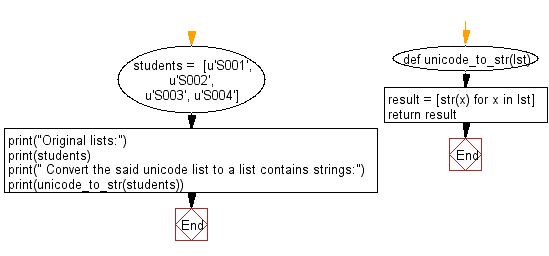﻿ Python: Convert a given unicode list to a list contains strings - w3resource# Python: Convert a given unicode list to a list contains strings

## Python List: Exercise - 199 with Solution

Write a Python program to convert a given unicode list to a list contains strings.

Sample Solution:

Python Code:

``````def unicode_to_str(lst):
result = [str(x) for x in lst]
return result
students =  [u'S001', u'S002', u'S003', u'S004']
print("Original lists:")
print(students)
print(" Convert the said unicode list to a list contains strings:")
print(unicode_to_str(students))
```
```

Sample Output:

```Original lists:
['S001', 'S002', 'S003', 'S004']
Convert the said unicode list to a list contains strings:
['S001', 'S002', 'S003', 'S004']
```

Flowchart:## Visualize Python code execution:

The following tool visualize what the computer is doing step-by-step as it executes the said program:

Python Code Editor:

Have another way to solve this solution? Contribute your code (and comments) through Disqus.

What is the difficulty level of this exercise?

Test your Python skills with w3resource's quiz

﻿

## Python: Tips of the Day

Floor Division:

When we speak of division we normally mean (/) float division operator, this will give a precise result in float format with decimals.

For a rounded integer result there is (//) floor division operator in Python. Floor division will only give integer results that are round numbers.

```print(1000 // 300)
print(1000 / 300)```

Output:

```3
3.3333333333333335```